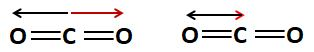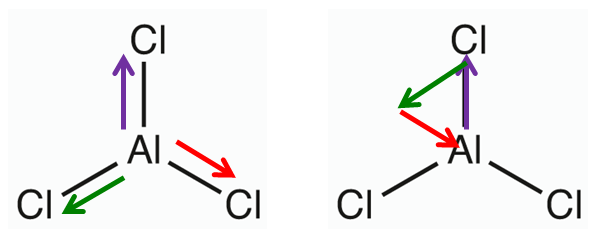# 8.8: Bond and Molecular Polarity

$$\newcommand{\vecs}{\overset { \rightharpoonup} {\mathbf{#1}} }$$ $$\newcommand{\vecd}{\overset{-\!-\!\rightharpoonup}{\vphantom{a}\smash {#1}}}$$$$\newcommand{\id}{\mathrm{id}}$$ $$\newcommand{\Span}{\mathrm{span}}$$ $$\newcommand{\kernel}{\mathrm{null}\,}$$ $$\newcommand{\range}{\mathrm{range}\,}$$ $$\newcommand{\RealPart}{\mathrm{Re}}$$ $$\newcommand{\ImaginaryPart}{\mathrm{Im}}$$ $$\newcommand{\Argument}{\mathrm{Arg}}$$ $$\newcommand{\norm}{\| #1 \|}$$ $$\newcommand{\inner}{\langle #1, #2 \rangle}$$ $$\newcommand{\Span}{\mathrm{span}}$$ $$\newcommand{\id}{\mathrm{id}}$$ $$\newcommand{\Span}{\mathrm{span}}$$ $$\newcommand{\kernel}{\mathrm{null}\,}$$ $$\newcommand{\range}{\mathrm{range}\,}$$ $$\newcommand{\RealPart}{\mathrm{Re}}$$ $$\newcommand{\ImaginaryPart}{\mathrm{Im}}$$ $$\newcommand{\Argument}{\mathrm{Arg}}$$ $$\newcommand{\norm}{\| #1 \|}$$ $$\newcommand{\inner}{\langle #1, #2 \rangle}$$ $$\newcommand{\Span}{\mathrm{span}}$$$$\newcommand{\AA}{\unicode[.8,0]{x212B}}$$

## Introduction

Carbon dioxide and water have molar masses of 44 amu and 18 amu respectively, and from common sense, we know that if you apply an equal force to two different objects of different masses, the lighter one moves faster. Common sense also tells us that a gas moves faster than a liquid, so why at ambient conditions (room temperature and room pressure) is the heavier carbon dioxide a gas while the lighter water is a liquid?

The answer to this seeming paradox is that although both water and carbon dioxide have polar bonds (Table $$\PageIndex{1}$$), only water is a polar molecule, and carbon dioxide is nonpolar because it is symmetric and its bond dipoles cancel. This can be visualized in Figure $$\PageIndex{1}$$,

Table $$\PageIndex{1}$$: $$\chi$$ and ($$\Delta \chi$$ data for water and carbon dioxide, showing that they both have polar bonds.
Atom Electronegativity ($$\chi$$) Bond ($$\Delta \chi$$) Molecule Dipole Moment
(D)
C 2.55 C-O 0.89 Water 1.85
O 3.44 O-H 1.44 Carbon Dioxide 0
H 2.20Figure $$\PageIndex{1}$$: Both carbon dioxide and water have polar bonds, but only water is a polar molecule. Carbon dioxide is symmetric and the pull of the two oxygens on the carbon's electrons cancel out, so it is a nonpolar molecule with polar bonds.

## Molecular Dipoles

The dipole moment of a molecule is the sum of all the bond dipoles within the molecule. These can be calculated by the following equation:

$\vec{\mu} = \sum_i q_i \, \vec{r}_i$

where

• $$\vec{\mu}$$ is the dipole moment vector
• $$q_i$$ is the magnitude of the $$i^{th}$$ charge, and
• $$\vec{r}_i$$ is the vector representing the position of $$i^{th}$$ charge.

For simple molecules we can use visualizations and a few tricks to determine if a molecule with polar bonds is polar or not. The First question we ask is, is it symmetric. If it is symmetric, than the bond dipoles cancel out and the molecule is non polar.

### Symmetric Geometries

In section 8.6 Molecular Geometries we learned that there are 5 VSPER geometries, which are indicated in Figure 8.8.2Figure $$\PageIndex{2}$$: Five Symmetric Geometries from VSEPR theory.

If we take a closer look we see there are only three geometries, linear, trigonal planar and tetrahedral, with trigonal bipyramidal being a combination of linear along the axis and trigonal planaar along the equatorial plan, and with octahedral being linear along each of the 3 Cartesian coordinate axes (x,y,z).

### Tip to Toe Technique for Vector Addition

One way to visualize the resultant vector from the addition of two (or more) vectors is to move the toe of the second vector to the tip of the first, with the resultant being the vector from the toe of the first to the tip of the second. In doing this, you can not change the orientation or the magnitude of the vector you moved. This is exemplified in Figure $$\PageIndex{3}$$.Figure $$\PageIndex{3}$$: Visualization of vector addition using the tip to toe technique. We want $$\vec{R}$$, the resultant from the addition of adding$$\vec{A}$$ to $$\vec{B}$$ in (a). In (b) we move the toe of $$\vec{A}$$ to the tip of $$\vec{B}$$, while in (c) we move the toe of $$\vec{B}$$ to the tip of $$\vec{A}$$. $$\vec{R}$$ is the same in either case (b) or (c).

Applying this technique to carbon dioxide shows the dipole moment is zeroFigure $$\PageIndex{4}$$: Here we show one of the C=O bonds in black and the other in red. When you move the toe of the red bond to the tip of the black, the resultant (toe of black to tip of red) is zero, as they are on top of each other.

Applying this technique to the trigonal planar structure of Aluminium chloride also creates a nonpolar molecule from the very polar Al-Cl bond $$\chi _{Al}$$= 1.61, $$\chi _{Cl}$$=3.16, so ($$\Delta \chi$$= 1.55, which is a highly polar bond. (Note: AlCl3 is a crystal in the solid phase, but a trigonal planar molecule in the vapor phase).Figure $$\PageIndex{5}$$: Here we show the three Al-Cl bonds in different colors, and when added, the resultant vector goes back to the central atom.

## Summary of Symmetric Structures

A=central atom, B,C,D = atoms of type B,C or D, E=Lone pair electrons

1. sp hybrid
• Linear
• AB2
2. sp2 hybrid
• Trigonal planar
• AB3
3. sp3 hybrid
• tetrahedral
• AB4
4. sp3d hybrid
• trigonal bipyramidal
• AB5
• AB2C3 (B in axial positions, C in equatorial plane)
• linear
• AB2E3 (B in axial positions, E - lone pairs in equatorial plane)
5. sp3d2 hybrid
• Octahedral
• AB6
• AB4C2 (B along X & Y axes, C along Z axis)
• AB2C2D2 (B along X, C along Y and D along Z axes)
• Square Planar
• AB4E2 (B along X & Y axes, E - lone pairs along Z axis)